Related Articles

# What is a Motion?

• Last Updated : 30 Jun, 2021

Kinematics is a branch of physics that deals with the objects in motion. It encompasses the study of the motion of small masses like points, objects, as well as a cluster of objects without taking into account the factors causing its motion, for instance, the forces applied over it. Kinematics can also be termed as the Geometry of Motion. Kinematics involves the evaluation of mathematical expressions, in order to study and analyze different aspects of motion such as velocity, acceleration, displacement, time, and trajectory.

The branch of physics concerned with the motion of material objects in consideration of the physical factors affecting them, that is force, mass, momentum, energy is known as the Dynamics.

Attention reader! All those who say programming isn't for kids, just haven't met the right mentors yet. Join the  Demo Class for First Step to Coding Course, specifically designed for students of class 8 to 12.

The students will get to learn more about the world of programming in these free classes which will definitely help them in making a wise career choice in the future.

### What is Motion?

The change in the position of anybody with respect to time can be termed as motion. Any object under motion can be visualized by the naked eye by determining the change in the positional coordinates and then, associating it through the eye of the arbitrary observer. Motion can be computed in terms of both the position vectors, that is, the displacement, distance, and taking into consideration the speed factors, that is, velocity, acceleration, speed, and time.

For instance, a spring ball attached to one end of a rod swinging at different time frames can be assumed to be in motion.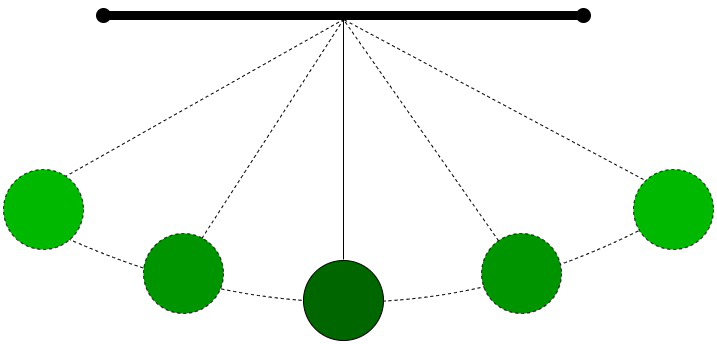A spring ball attached to a rod.

### Kinematics of a Particle Trajectory

The trajectory is referred to as the curved path followed by an object with respect to its motion in consideration of gravity. It can also be termed projectile motion. Kinematic equations are used to analyze and effectively compute the trajectory followed by particles or objects. The trajectory computations involve the derivations using physical quantities considered relevant to the motion of a particle including, mass m, position r, velocity v, acceleration a.

For instance, an object of mass m moving with distance r witnesses a change in distance referred to as dr. Therefore, velocity (v) is referred to as the change in distance with respect to time and is given by:

v = dr / dt

And acceleration (a) is referred to as the change in velocity of an object with respect to time. Acceleration may be positive, negative, or even zero and is given by:

a = dv / dt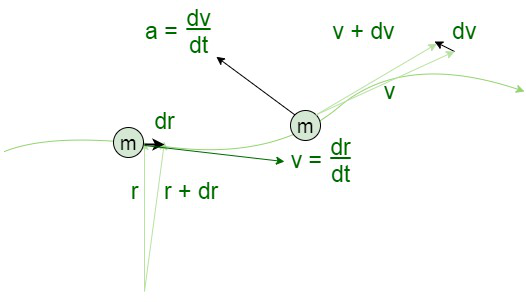Kinematics of a Particle Trajectory.

Rest: Any object is said to be at a rest position if there is no change in its position (distance, displacement) with time, with respect to a reference point. The body does not undergo any modification in its position, orientation and pattern when in a state of rest.

### Factors affecting the Motion of an Object

There are four main factors that affect the motion of objects listed and discussed below:

1. Distance (d)
2. Displacement (s)
3. Speed (v)
4. Time (t)

### Distance

Distance is used to refer to the complete path length between any two successive points. The distance is a scalar quantity, with only magnitude and no associated direction. Therefore, the distance is always positive in nature. Distance of a body gives the descriptive route information being followed by an object from one point to another. Since the distance between two points is equivalent to the path length, it can be measured across different trajectories, that is, linear or zigzag paths. The distance is denoted by the symbol ‘d’.

Distance = Speed × Time

or

d = v × t

For instance, the distance between points A and B is 5 cm in the below figure.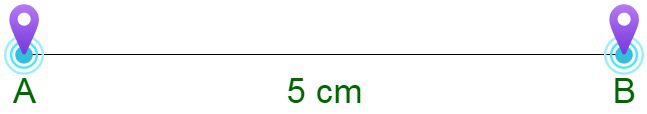Distance between points A and B.

### Displacement

Displacement is the direct length of the minimum path between any two successive points. Displacement, therefore, may refer to the displacement as a vector quantity, with both an associated magnitude and direction. The displacement of an object between any two points is considered to be positive, negative, and even zero. Displacement is independent of the path and only depends upon the initial and final position of the body. Therefore, it does not provide complete information on the route. Displacement is always indicated with an arrow.  It is denoted by ‘s’.

Displacement = Velocity × Time

or

s = v × t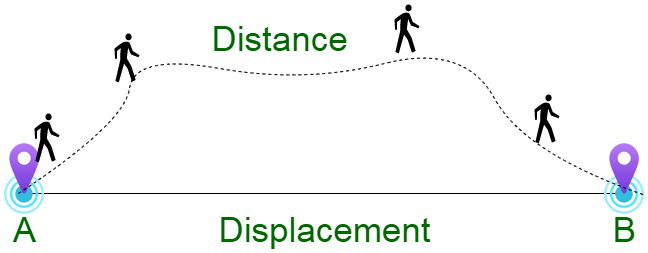Displacement between points A and B.

### Speed

Speed can be defined as the rate of change of position of an object moving in any direction. Speed is measured as the ratio of the distance covered by an object to the total time to cover this distance. Speed of a body is considered to be a scalar quantity, with only magnitude and no associated direction.

Speed = Distance / Time

or

v = d / t

The unit of Speed in different system is different as shown below:

The Dimensional formula for Speed is [ML1T-1].

### Time

Time is an important frame of reference to evaluate the change in state and motion of the objects. Time is referred to as the interval over which any object undergoes modifications in its motion, orientation and structure. The criteria for evaluation that time has passed is the modification in any object. The S.I. unit for time is the second, abbreviated s.

Time and motion are interdependent on each other. All moving bodies change their position and orientation with time. Time and motion are coexisting concepts. Without time, there would be no change. And without any motion in objects, the time could not be visualized. No concept of time can exist without motion. Therefore, the amount of time change is calibrated by comparison with a standard.

For instance, if we consider a wristwatch, it has three hands: a seconds’ hand, a minutes’ hand, and a hours’ hand, which moves around the dial. The second-hand moves quickly, and therefore, its movement is easily noticeable. The hours’ and minutes’ hand movement is not easily visible without concentration. It is visible a few times. This is because when a body moves, its position changes with time.

The Dimensional formula for time is [M0L0T1].

### Types of Motion

As per physics and mechanics, there are mainly four categories of motion, i.e.

1. Rotary Motion
2. Oscillatory Motion
3. Linear Motion
4. Reciprocating Motion

### Rotary Motion

The type of motion where the object witnesses rotation around a fixed axis like a figure skater rotating on an ice rink. This takes into consideration the dynamics of rigid bodies, that is the objects with a definite mass and holding a rigid shape. The radius of motion of the object doesn’t change in this scenario. The rotational motion is completely analogous to linear or translational dynamics. Most of the equations for mechanics of rotating objects are considered to be equivalent to the motion equations for linear motion.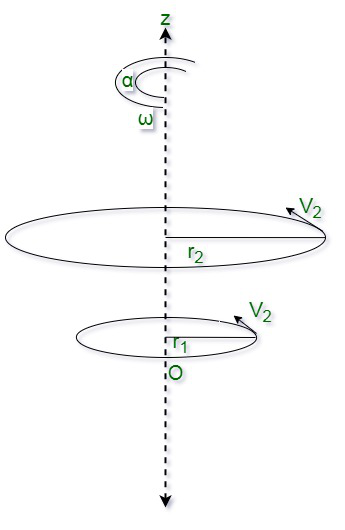Rotational Motion about a fixed axis

Examples :

• Rotation of the minute hand and the hour hand in the clock
• Ceiling fan rotation
• Opening and closing of the door

### Oscillatory Motion

Oscillatory motion is a kind of repetitive motion, which occurs when the object repeats the same movement again and again. The body in consideration keeps on swinging to and fro about its mean position. Oscillatory motion puts an object into constant motion. However, the presence of frictional and balancing forces eventually stops the motion of the objects by bringing them to a state of equilibrium.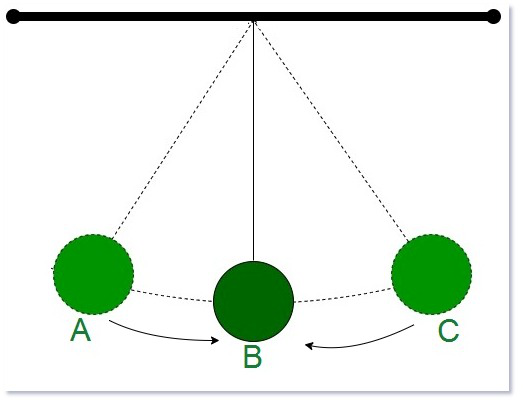Oscillatory Motion of a pendulum

Examples:

• A swinging swing
• The motion of a pendulum
• A boat tossing up and down a river
• The tuning fork

### Linear Motion

Linear motion is the motion that is natural to an object: moving in a straight line. According to Newton s First Law of Motion, an object not affected by any force will continue indefinitely in a straight line. If a projectile is thrown vertically, it will travel in linear motion and will begin to fall when the force of gravity equals the force of the throw.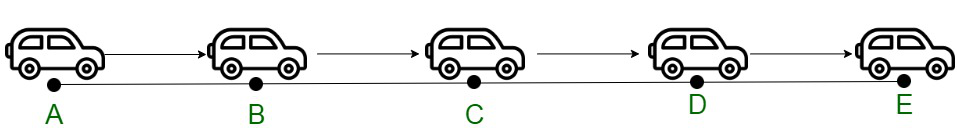Car moving on a straight path

Example:

• An athlete running 100m along a straight track.
• A car moving on a straight road.

The linear motion depending on the path of motion is further divided as follows

(1) Rectilinear Motion: When we require only one co-ordinate axis along with time to describe the motion of a particle it is said to be in linear motion or rectilinear motion. Some examples of linear motion are a parade of soldiers, a train moving along a straight line, and many more.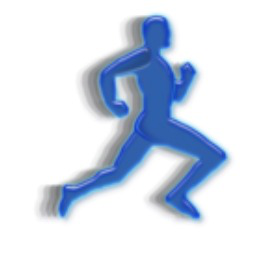Racer running in a rectilinear Motion

Examples:

• The use of elevators in public places is an example of rectilinear motion.
• Gravitational forces acting on objects resulting in free fall is an example of rectilinear motion.
• Kids sliding down from a slide is a rectilinear motion.
• The motion of planes in the sky is a rectilinear motion.

(2) Curvilinear Motion: Curvilinear motion is defined as motion that occurs when a particle travels along a curved path. The curved path can be in two dimensions (in a plane), or in three dimensions. This type of motion is more complex than rectilinear (straight-line) motion.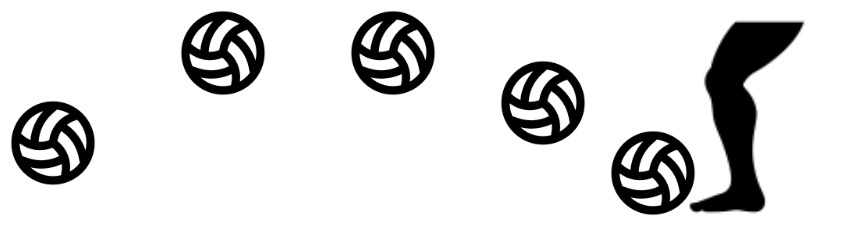Ball falling on the ground in Curvilinear Motion

Examples:

• Throwing the ball into the air in a curved way,
• The motion of a jet in a curved path
• Turning a car
• Planets revolving around the orbit

### Reciprocating Motion

Reciprocating motion, also termed reciprocation, is a repetitive up-and-down or back-and-forth linear motion. Each pair of opposite motions comprising of a single reciprocation cycle are called strokes. Circular motion can be easily transformed into a reciprocating motion and vice versa, using a crank.

Examples:

• Internal combustion in engines and pumps
• Burning fuel’s expansion in the cylinders
• Movement in loudspeaker coil
• Needle’s movement in a tailoring machine
• Electric bell’s ringer.

Types of Motion as per State of Motion are:

1. Uniform Motion
2. Non-Uniform Motion

### Uniform Motion

The uni-dimensional motion of an object where it travels with uniform speed all along the path is called Uniform motion. Since the body covers equal distances in equal intervals of time, the velocity of the body remains constant. The speed of an object remains the same during all time frames, the average speed of the object is equivalent to its actual speed. No acceleration is attained by the object in the case of uniform motion. For instance, a car traveling 20 km in the first hour, 20 km in the next hour, and so on continuing with this throughout its motion.

Examples:

• The hands of the clock covering equal distances.
• A car going along a straight level road at a steady speed.
• An airplane flying at a steady speed in the air.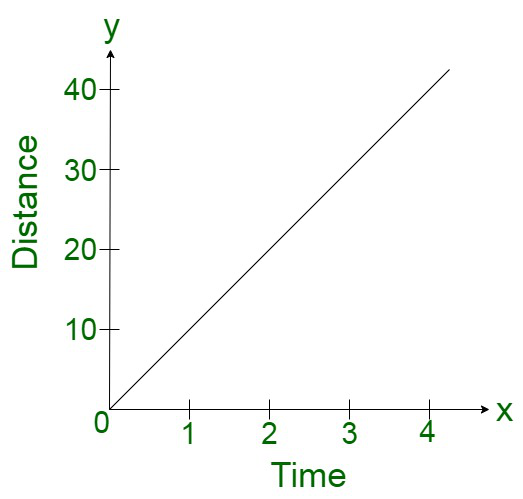Distance Time Graph for Uniform Motion

### Non-Uniform Motion

The uni-dimensional motion of an object where it travels with a varying speed all along the path is called Non-Uniform motion. Since the body covers unequal distances in equal intervals of time, the velocity of the body remains modified. The speed of an object changes during the time frames, the average speed may be different from its actual speed. Acceleration or deceleration is attained by the object in the case of non-uniform motion. For instance, a car traveling 20 km in the first hour, 30 km in the next hour, and so on. Continuing with a varying speed throughout its motion.

Examples:

• A racing person.
• A ball bouncing at different intervals
• Two cars colliding with each other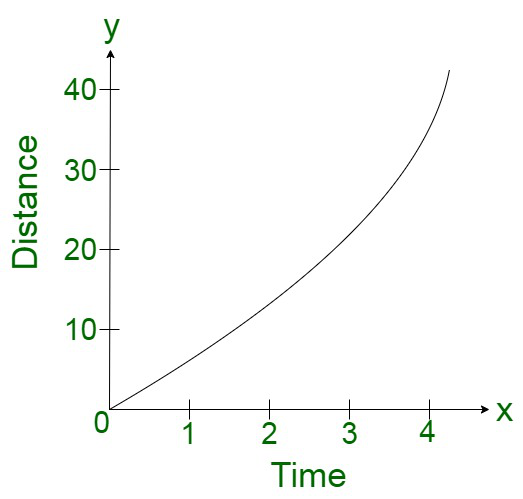Distance Time Graph for Non-Uniform Motion

Types of Motion as Per Directions are:

1. One Dimensional Motion
2. Two Dimensional Motion
3. Three Dimensional Motion

### One Dimensional Motion

Whenever anyone out of the three coordinate spaces representing the position of the object undergoes any change (shape, speed, distance) with respect to time, then that motion is called one-dimensional motion, or uni-dimensional motion.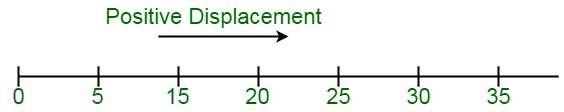One Dimensional Motion – change in x coordinate

Examples:

• The motion of a steel block in a straight line,
• Object freely falling under the effect of gravity.
• A man walking through a straight lane

### Two Dimensional Motion

Whenever any pair out of the three coordinate spaces representing the position of the object undergoes any change (shape, speed, distance) with respect to time, then that motion is called two-dimensional motion, or bi-dimensional motion.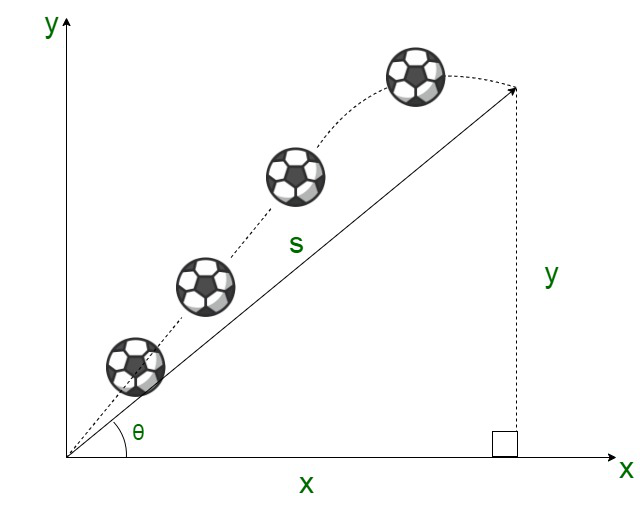Two Dimensional Motion, change in both x and y coordinates

Examples :

• Movement of a train along a zigzag track.
• The rotation of the planets around the sun

### Three Dimensional Motion

Whenever all the three coordinate spaces representing the position of the object undergoes any change (shape, speed, distance) with respect to time, then that motion is called three-dimensional motion. The body tends to undergo movement within a planar structure.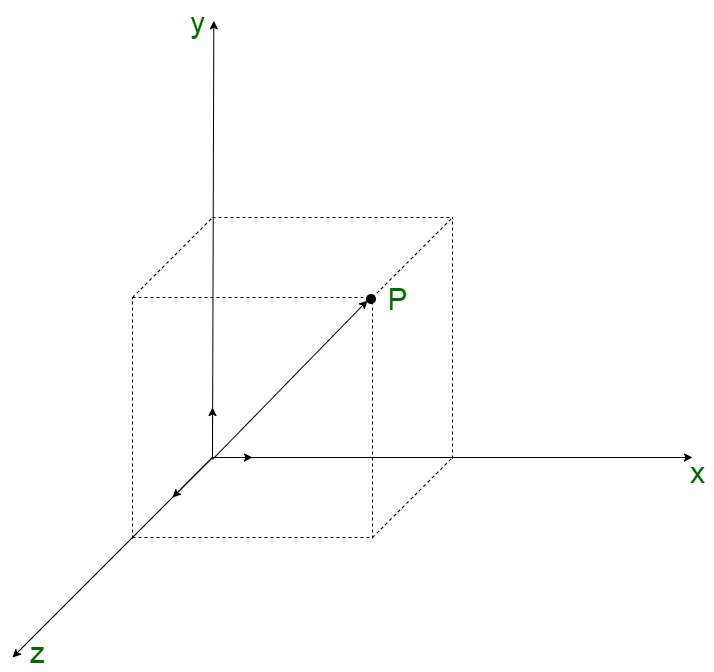Examples :

• Objects flying in arbitrary paths in the sky.
• The motion of atoms inside a gas molecule.

### Sample Problems

Problem 1: Define translation motion.

Solution:

Translational motion is the motion by which a body shifts from one point in space to another. Translational motion encompasses both rectilinear and curvilinear motion of the objects. Every component of the body moves through the same portion in case of these motions. For instance, a bullet fired through a gun.

Problem 2: Differentiate between periodic and non-periodic motion.

Solution:

The difference between periodic and non-periodic motion are:

Problem 3. Differentiate between Curvilinear Motion and Rectilinear Motion?

Solution:

The difference between Curvilinear Motion and Rectilinear Motion are:

Problem 4: Can the motion be absolute?

Solutions :

Motion is described in terms of speed, displacement, time, and acceleration. Since there is no absolute frame of reference, therefore the estimation of motion can’t be absolute.

Problem 5. A person A travels from Delhi to Mumbai at a speed of 30 km/hr and returns at an increasing speed of 120 km/hr in 5 hours. Compute the total distance traveled by person A.

Solutions:

Since, we know,

Distance traveled is constant.

Therefore,

Time taken will be inversely proportional to the Speed.

The ratio of speeds is given as 30:120, i.e. 1: 4

Therefore,

The ratio of time taken will be opposite, in the ratio of 4: 1.

Now,

Total Time taken = 5 hours.

Hence, Distance = 30 × 4 = 120 km

My Personal Notes arrow_drop_up